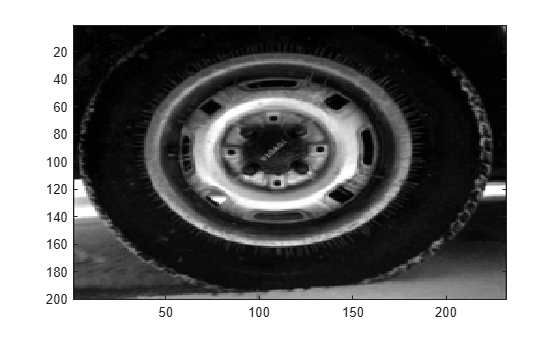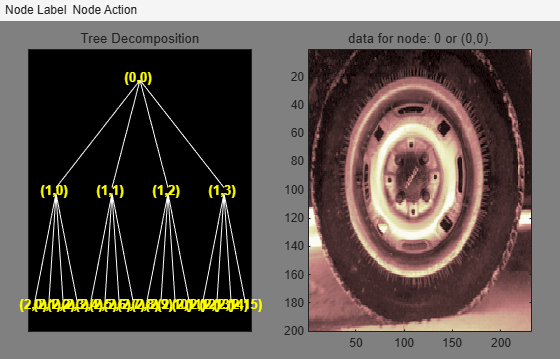# wpdec2

Wavelet packet decomposition 2-D

## Syntax

``T = wpdec2(X,N,wname)``
``T = wpdec2(X,N,wname,E,P)``

## Description

example

````T = wpdec2(X,N,wname)` returns a wavelet packet tree `T` corresponding to the wavelet packet decomposition of the matrix `X`, at level `N`, with the specified wavelet `wname` using Shannon entropy. Note`T = wpdec2(X,N,wname)` is equivalent to ```T = wpdec2(X,N,wname,'shannon')```. ```
````T = wpdec2(X,N,wname,E,P)` uses the entropy type specified by `E`. `P` is an optional parameter depending on the value of `E`. See `wentropy` for more information.```

## Examples

collapse all

```load tire image(X) colormap(map)```Obtain the 2-level wavelet packet decomposition of the image. Use the Haar wavelet. The default entropy is `shannon`.

`t = wpdec2(X,2,"haar");`

Plot the wavelet packet tree.

`plot(t)`## Input Arguments

collapse all

Input data, specified as a matrix.

Data Types: `double`

Decomposition level, specified as a positive integer.

Data Types: `double`

Wavelet used in the wavelet packet decomposition, specified as a character vector or string scalar. The wavelet is from one of the following wavelet families: Best-localized Daubechies, Beylkin, Coiflets, Daubechies, Fejér-Korovkin, Haar, Han linear-phase moments, Morris minimum-bandwidth, Symlets, Vaidyanathan, Discrete Meyer, Biorthogonal, and Reverse Biorthogonal. See `wfilters` for the wavelets available in each family.

Entropy type, specified as one of the following:

Entropy Type (`T`)

Threshold Parameter (`P`)

`'shannon'`

`P` is not used.

`'log energy'`

`P` is not used.

`'threshold'``0 ≤ P`

`P` is the threshold.

`'sure'``0 ≤ P`

`P` is the threshold.

`'norm'``1 ≤ P`

`P` is the power.

`'user'`Character vector

`P` is a character vector containing the file name of your own entropy function, with a single input `X`.

'`FunName`'No constraints on `P`

`FunName` is any character vector other than the previous entropy types listed.

`FunName` contains the file name of your own entropy function, with `X` as input and `P` as an additional parameter to your entropy function.

`T` and the threshold parameter `P` together define the entropy criterion. See `wentropy` for more information.

Note

The `'user'` option is historical and still kept for compatibility, but it is obsoleted by the last option described in the table above. The FunName option does the same as the `'user'` option and in addition gives the possibility to pass a parameter to your own entropy function.

Threshold parameter, specified by a real number, character vector, or string scalar. `P` and the entropy type `E` together define the entropy criterion.

collapse all

### Wavelet Packet Decomposition

The wavelet packet method is a generalization of wavelet decomposition that offers a richer signal analysis. Wavelet packet atoms are waveforms indexed by three naturally interpreted parameters: position and scale as in wavelet decomposition, and frequency.

For a given orthogonal wavelet function, a library of wavelet packets bases is generated. Each of these bases offers a particular way of coding signals, preserving global energy and reconstructing exact features. The wavelet packets can then be used for numerous expansions of a given signal.

Simple and efficient algorithms exist for both wavelet packets decomposition and optimal decomposition selection. Adaptive filtering algorithms with direct applications in optimal signal coding and data compression can then be produced.

In the orthogonal wavelet decomposition procedure, the generic step splits the approximation coefficients into two parts. After splitting we obtain a vector of approximation coefficients and a vector of detail coefficients, both at a coarser scale. The information lost between two successive approximations is captured in the detail coefficients. The next step consists in splitting the new approximation coefficient vector; successive details are never re-analyzed.

In the corresponding wavelet packets situation, each detail coefficient vector is also decomposed into two parts using the same approach as in approximation vector splitting. This offers the richest analysis: the complete binary tree is produced in the one-dimensional case or a quaternary tree in the two-dimensional case.

## Tips

• When X represents an indexed image, X is an `m`-by-`n` matrix. When X represents a truecolor image, it is an `m`-by-`n`-by-3 array, where each `m`-by-`n` matrix represents a red, green, or blue color plane concatenated along the third dimension.

For more information on image formats, see `image` and `imfinfo`.

## Algorithms

The algorithm used for the wavelet packets decomposition follows the same line as the wavelet decomposition process (see `dwt2` and `wavedec2` for more information).

 Coifman, R.R., and M.V. Wickerhauser. “Entropy-Based Algorithms for Best Basis Selection.” IEEE Transactions on Information Theory 38, no. 2 (March 1992): 713–18. https://doi.org/10.1109/18.119732.

 Jaffard, Stéphane, Yves Meyer, and Robert Dean Ryan. Wavelets: Tools for Science & Technology. Philadelphia: Society for Industrial and Applied Mathematics, 2001.

 Wickerhauser, Mladen Victor. “INRIA lectures on wavelet packet algorithms,” Proceedings ondelettes et paquets d'ondes, 17–21 June 1991, Rocquencourt, France, pp. 31–99.

 Wickerhauser, Mladen Victor. Adapted Wavelet Analysis from Theory to Software. Wellesley, MA: A.K. Peters, 1994.